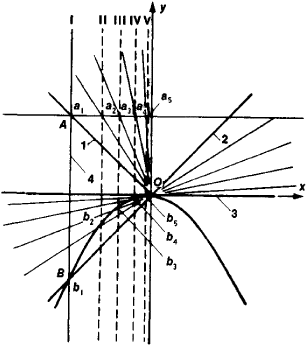# Parabolograph

The following article is from The Great Soviet Encyclopedia (1979). It might be outdated or ideologically biased.

## Parabolograph

an instrument for drawing plane curves of the second order—parabolas. Its operation is based on plotting the points of the parabola in a rectangular coordinate system. Figure 1 is a schematic representation of a simple parabolograph and of its mode of operation. The instrument consists of a guide, represented by the x-axis; a rod that can slide along the guide but remains parallel to the y-axis; and a pair of rigidly connected and mutually perpendicular straightedges that can rotate about O. A shows the position of a fixed pin on the rod, and B the corresponding position of a sliding pen on straightedge 2. As the rod slides along the guide, it forces the joined straightedges to rotate about O. Then the pen rotates about O and slides along straightedge 2. The curve traced by the pen is a parabola. Parabolographs enable us to draw parabolas without the use of french curves.

D. N. OSIPOVFigure 1. Schematic of a parabolograph: O is the axis of rotation; A and B are the points of connection between the straightedges 1 and 2 and the rod 4; 3 is the guide; χ and y are the coordinate axes of the plane